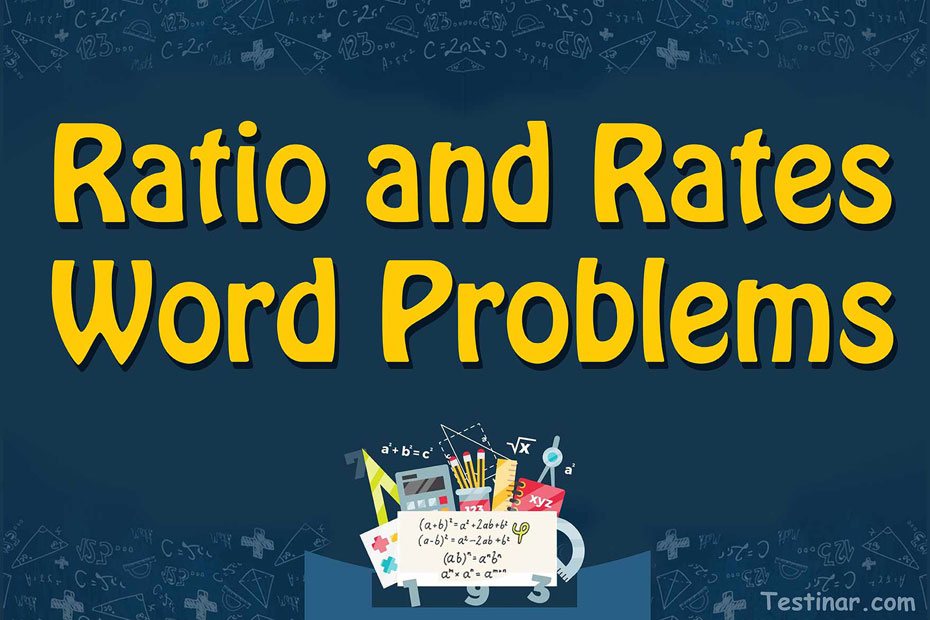## How to write ratios in a word problem

In mathematics, a ratio is defined as the comparison between two numbers. This is generally done to find out how big or small a number or a quantity is with respect to another. So, what method do we use to find these ratios? Well, we use the division method. In a ratio, two numbers are divided. The dividend part is known as the ‘antecedent’, whereas the divisor is known as the ‘consequent.’
So, let’s look at an example. Suppose, in a market of $$40$$ vendors, $$23$$ of them sell apples, and the rest $$17$$ sell bananas. So, the ratio of the vendors selling apples to those selling bananas would be $$23 \ : \ 17$$. Moreover, in mathematical terms, this is read as “$$23$$ is to $$17$$.”

### Types of Ratios

Now, there are $$2$$ types of ratios. They are called “part-to-part” and “part-to-whole.” So, when we say that in a group of $$17$$ teachers, $$9$$ teach math and $$8$$ teach physics, then the part-to-part ratio is $$9 \ : \ 8$$. Basically, a part-to-part ratio denotes the clear distinction between two entities.
Now, if we say that out of $$10$$ books in a library, $$7$$ are of computer science. So, here part-to-whole ratio is $$7 \ : \ 10$$. This means that out of $$10$$ books in the library, every $$7$$ are of computer science. So, basically, a part-to-whole ratio denotes the ratio between the whole group to a specific entity.

### Calculation of Ratios

To understand the exact process of calculating ratios, let’s take up the following problem.
Question: Suppose, $$24$$ tigers and $$17$$ lions make up for an entire zoo. So, what is the ratio of tigers and lions in that zoo?

• Firstly, identify the unique entities. In this case, $$24$$ tigers and $$17$$ lions are unique entities.
• Next, write these in a fraction form. So, we write it as $$\frac{24}{17}$$
• Now, we need to check if this fraction can be further simplified or not. Here, it can’t be simplified further.
• So, the ratio of tigers and lions in the zoo is $$24 \ : \ 17$$.

### Simplifying Ratios

Check the entities in the given problem for writing ratios. Then divide both sides of the ratio to simplify it further. Suppose there are $$14$$ apples and $$7$$ oranges. So, the ratio of apples to oranges would be $$\frac{14}{7} \ = \ 14 \ : \ 7 \ = \ 2 \ : \ 1$$.

### Ratio and Rates Word Problems

Now suppose, a problem like this is given, where $$x \ : \ 4 \ = \ 4 \ : \ 16$$ and we are required to find $$x$$.
So, we can write, $$\frac{x}{4} \ = \ \frac{4}{16}$$ and by cross multiplication and simplifying the fractions, we can get $$x=1$$.

## Ratio and Rates Word Problems Quiz

### SAT Math Test Prep Bundle

$69.99$35.99

### TABE Math Practice Workbook

$25.99$14.99

### CHSPE Math for Beginners 2022

$24.99$14.99

### GED Math in 10 Days

$26.99$13.99